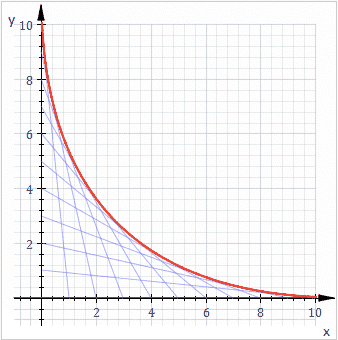# Can an envelope curve cut its member curve twice?

• I
Can the envelope curve (of a family of curves) intersect a member curve of the family at more than one point?

It seems possible. Consider the following.Each blue line is a member curve and the red line is the envelope curve. If we modify each blue line such that it has a protrusion like a "þ", then it can intersect the red line more than once and still has a point that is tangent to the red line.

But I have never seen such as example before. So is it not allowed?

Picture from https://en.wikipedia.org/wiki/Envelope_(mathematics)

mfb
Mentor
You would get a different envelope, being tangent to the "þ". You can probably make multiple envelope curves then.

•Happiness
jbriggs444
Homework Helper
Consider the envelope defined by ##f(x) = 1## for the family of curves ##g_k(x) = sin(x+k)##

That envelope is tangent to each family member at infinitely many points. My understanding of an "envelope" is that it is not permissible for a family member to extend beyond the envelope. You are only allowed to kiss, not penetrate.

My understanding of an "envelope" is that it is not permissible for a family member to extend beyond the envelope. You are only allowed to kiss, not penetrate.

This would go well with the English meaning of the word envelope.

But it is not required in the following definition: an envelope of a family of curves in a plane is a curve that is tangent to each member of the family at some point.

•jbriggs444